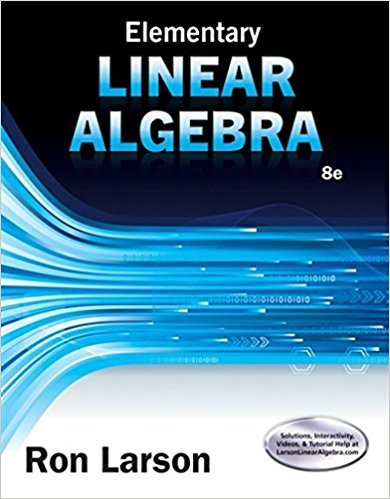×
×

# Solutions for Chapter 6-7: Cumulative Test## Full solutions for Elementary Linear Algebra | 8th Edition

ISBN: 9781305658004Solutions for Chapter 6-7: Cumulative Test

Solutions for Chapter 6-7
4 5 0 404 Reviews
18
0
##### ISBN: 9781305658004

This expansive textbook survival guide covers the following chapters and their solutions. Since 32 problems in chapter 6-7: Cumulative Test have been answered, more than 44312 students have viewed full step-by-step solutions from this chapter. Elementary Linear Algebra was written by and is associated to the ISBN: 9781305658004. This textbook survival guide was created for the textbook: Elementary Linear Algebra, edition: 8. Chapter 6-7: Cumulative Test includes 32 full step-by-step solutions.

Key Math Terms and definitions covered in this textbook
• Adjacency matrix of a graph.

Square matrix with aij = 1 when there is an edge from node i to node j; otherwise aij = O. A = AT when edges go both ways (undirected). Adjacency matrix of a graph. Square matrix with aij = 1 when there is an edge from node i to node j; otherwise aij = O. A = AT when edges go both ways (undirected).

• Cayley-Hamilton Theorem.

peA) = det(A - AI) has peA) = zero matrix.

• Characteristic equation det(A - AI) = O.

The n roots are the eigenvalues of A.

• Companion matrix.

Put CI, ... ,Cn in row n and put n - 1 ones just above the main diagonal. Then det(A - AI) = ±(CI + c2A + C3A 2 + .•. + cnA n-l - An).

• Cramer's Rule for Ax = b.

B j has b replacing column j of A; x j = det B j I det A

• Diagonalization

A = S-1 AS. A = eigenvalue matrix and S = eigenvector matrix of A. A must have n independent eigenvectors to make S invertible. All Ak = SA k S-I.

• Distributive Law

A(B + C) = AB + AC. Add then multiply, or mUltiply then add.

• Elimination matrix = Elementary matrix Eij.

The identity matrix with an extra -eij in the i, j entry (i #- j). Then Eij A subtracts eij times row j of A from row i.

• Jordan form 1 = M- 1 AM.

If A has s independent eigenvectors, its "generalized" eigenvector matrix M gives 1 = diag(lt, ... , 1s). The block his Akh +Nk where Nk has 1 's on diagonall. Each block has one eigenvalue Ak and one eigenvector.

• Kirchhoff's Laws.

Current Law: net current (in minus out) is zero at each node. Voltage Law: Potential differences (voltage drops) add to zero around any closed loop.

• Left inverse A+.

If A has full column rank n, then A+ = (AT A)-I AT has A+ A = In.

• Length II x II.

Square root of x T x (Pythagoras in n dimensions).

• Norm

IIA II. The ".e 2 norm" of A is the maximum ratio II Ax II/l1x II = O"max· Then II Ax II < IIAllllxll and IIABII < IIAIIIIBII and IIA + BII < IIAII + IIBII. Frobenius norm IIAII} = L La~. The.e 1 and.e oo norms are largest column and row sums of laij I.

• Normal equation AT Ax = ATb.

Gives the least squares solution to Ax = b if A has full rank n (independent columns). The equation says that (columns of A)·(b - Ax) = o.

• Reduced row echelon form R = rref(A).

Pivots = 1; zeros above and below pivots; the r nonzero rows of R give a basis for the row space of A.

• Rotation matrix

R = [~ CS ] rotates the plane by () and R- 1 = RT rotates back by -(). Eigenvalues are eiO and e-iO , eigenvectors are (1, ±i). c, s = cos (), sin ().

• Singular matrix A.

A square matrix that has no inverse: det(A) = o.

• Standard basis for Rn.

Columns of n by n identity matrix (written i ,j ,k in R3).

• Subspace S of V.

Any vector space inside V, including V and Z = {zero vector only}.

• Vector space V.

Set of vectors such that all combinations cv + d w remain within V. Eight required rules are given in Section 3.1 for scalars c, d and vectors v, w.

×